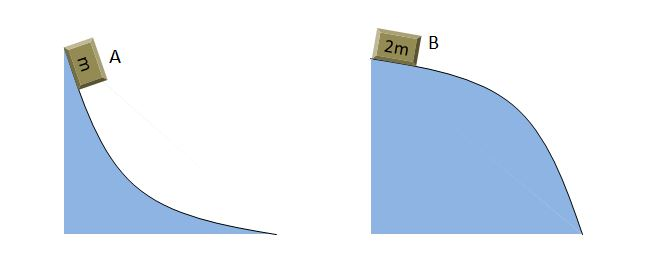# Potential Energy of the boxesBox A and B lie on differently curved surfaces as above. The masses of box A and B are $m$ and $2m$, respectively, and the heights and lengths of the curved surfaces are the same. Which of the following statements is correct? (The frictions on the curved surfaces and the air resistance are negligible. And suppose the boxes are particles.)

a) The average velocity of A until the box arrives at the bottom is greater than that of B.

b) The works done by gravity on the boxes A and B are the same.

c) The mechanical energies at the moment both A and B arrive at the bottom are the same.

×